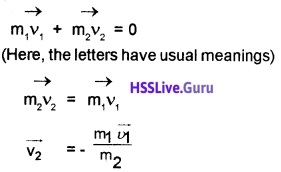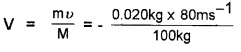# Plus One Physics Chapter Wise Questions and Answers Chapter 5 Law of Motion

Students can Download Chapter 5 Law of Motion Questions and Answers, Plus One Physics Chapter Wise Questions and Answers helps you to revise the complete Kerala State Syllabus and score more marks in your examinations.

## Kerala Plus One Physics Chapter Wise Questions and Answers Chapter 5 Law of Motion

### Plus One Physics Law of Motion One Mark Questions and Answers

Plus One Physics Laws Of Motion Questions Chapter 5 Question 1.
Which one of the following is not a force?
(a) Impulse
(b) Tension
(c) Thrust
(d) Weight
(a) Impulse
Tension, thrust, weight are all common forces in mechanics whereas impulse is not a force.
Impulse = Force × Time duration.

Plus One Physics Laws Of Motion Questions And Answers Chapter 5 Question 2.
A passenger getting down from a moving bus, falls in the direction of the motion of the bus. This is an example for
(a) Inertia of motion
(b) Second law of motion
(c) Third law of motion
(d) Inertia of rest
(a) Inertia of motion
A passenger getting down from a moving bus, falls in the direction of the motion of the bus. This is because his feet come to rest on touching the ground and the remaining body continues to move due to inertia of motion.

Plus One Physics Important Questions And Answers Pdf Chapter 5  Question 3.
Which one of the following is not a contact force?
(a) Viscous force
(b) Magnetic force
(c) Friction
(d) Buoyant force
(b) Magnetic force

Plus One Physics Chapter Wise Questions And Answers Chapter 5 Question 4.
A jet engine works on the principle of
(a) Conservation of linear momentum
(b) Conservation of mass
(c) Conservation of energy
(d) Conservation of angular momentum
(a) Conservation of linear momentum
A jet engine works on the principle of linear momentum.

State And Prove Impulse Momentum Theorem Chapter 5 Question 5.
Newton’s second and third laws of motion lead to the conservation of
(a) linear momentum
(b) angular momentum
(c) potential energy
(d) kinetic energy
(a) linear momentum
Newton’s second and third laws lead to the conservation of linear momentum.

Hsslive Plus One Physics Chapter Wise Questions And Answers Chapter 5 Question 6.
A large force is acting on a body for a short time. The impulse imparted is equal to the change in
(a) acceleration
(b) momentum
(c) energy
(d) velocity
(b) momentum
If a large force F acts for a short time dt, the impulse imparted is
I = F.dt, = $$\frac{d p}{d t}$$.dt
I = dp = change in momentum.

Laws Of Motion Class 11 Questions With Solutions Pdf Chapter 5 Question 7.
When a shell explodes, the fragments fly apart though no external force is acting on it. Does this violate Newton’s first law of motion?
No. The explosion takes place due to the internal force. The internal force does not change the position of centre of mass.

Prove Impulse Momentum Theorem Chapter 5 Question 8.
In taking a catch, a cricket player moves his hands backward on holding the ball. Why?
We know F = $$\frac{\Delta P}{\Delta t}$$
When ∆t increases, the force acting on hand decreases.

350 degrees f to c … T C T F 32 x 59 is the method for converting degrees Fahrenheit to degrees Celsius.

Plus One Physics Important Questions And Answers Chapter 5 Question 9.
Name the factor on which inertia depends.
Mass

Laws Of Motion Class 11 Test Paper Chapter 5 Question 10.
Why does a swimmer push the water backwards?
A swimmer pushes the water backward in order to be pushed forward (Newton’s third law).

Laws Of Motion Previous Year Questions Chapter 5 Question 11.
Rocket works on the principle of conservation of_______.
Momentum

Motion Questions And Answers Pdf Chapter 5 Question 12.
A man experience a backward jerk, while firing bullet from gun. Which law is applicable here? Answer:
Conservation of momentum.

Plus One Physics Laws Of Motion Notes Chapter 5 Question 13.
If you jerk a piece of paper under a book quick enough, the book will not move. Why?
This is due to inertia of rest.

Class 11 Physics Chapter 5 Important Questions Chapter 5 Question 14.
Why it is difficult to walk on a slipper road?
We will not get required reaction from slippery road.

Laws Of Motion Class 11 Important Questions Chapter 5 Question 15.
A stone, when thrown on a glass window, smashes the window pan to pieces. But a bullet fired from the gun passes through it making a hole why?
This is due to inertia of rest of glass window.

Question 16.
Why an athlete runs some distance before taking a jump?
An athletic runs some distance before taking a jump to gain some initial momentum. It helps the athlete to jump more.

Question 17.
Why a horse can not pull a cart and run in empty space?
The horse-cart system moves forward due to reaction of ground on the feet of horse. In free space, there is no reaction. So it can not pull cart.

Question 18.
Why parachute descends slowly?
Parachute has large surface area. This increases fluid friction and slows down the motion of parachute.

Question 19.
Sand is thrown on tracks with snow. Why?
The presence of snow on tracks reduces friction and driving is not safe. If sand is thrown, friction will be increased and driving becomes safe.

Question 20.
It is difficult to move a cycle along a road with its brakes on. Explain.
When the cycle is moved with its brakes on, wheels can only skid. There will be sliding friction. The sliding friction is more compared to rolling friction. Hence it is difficult to move a cycle with its brakes on.

### Plus One Physics Law of Motion Two Mark Questions and Answers

Question 1.
Two masses are in the ratio 1:5

1. What is inertia.
2. What is the ratio of inertia of above case?

1. The inability of a body to change it’s state of rest or uniform motion is called inertia.
2. Mass is a measure of inertia. Hence ratio of inertia is 1:5.

Question 2.
More force is required to push a body than pull to get same speed on a ground with some friction. Why?
When we push, the action on the surface and normal reaction on the body increases. (Friction is directly proportional to normal reaction).

As a result more force is required to push the body. When we pull, normal reaction decreases. Hence friction decreases. Hence less force is required to pull the body.

Question 3.
A lift in a multistoried building is moving from ground floor to third floor. What will happen to weight of a person sitting in side of the lift.

1. A When starts to move up from ground floor.
2. When the lift moves with constant speed.

1. A weight increases weight w = mg + ma
2. weight is constant ie. w = mg

Question 4.
Why it is advisable to hold a gun tight to one’s shoulder when it is being fired?
The recoiling gun can hurt the shoulder. If gun is held tightly against the shoulder, the body and gun act a system. This will reduce recoil velocity as it is inversly proportional to mass of system.

Question 5.
Why shockers are used in vehicles?
When there is a jerk or jump, the time for which force acts (∆t) increases. As the product of force and time for which force acts (F∆t) remains constant, increase in At will reduce the force. This provide smooth motion.

### Plus One Physics Law of Motion Three Mark Questions and Answers

Question 1.
Give the magnitude and direction of net force on

1. a drop of rain falling down with a constant velocity.
2. a stone of mass 0.1 kg just after it dropped from the window of a tram accelerating at 1 ms-2.

1. Net force is zero

2. When stone is dropped, gravitational force will act on the stone.
Gravitational force F = mg
= 0.1 × 10
= 1 N downward.

Question 2.
An external force is always required to break the inertia of a body which is either in the state of rest or state of uniform motion.

1. Which law governs this statement?
2. Can all forces produce acceleration? Why?
3. A boy holding a spring balance in his hand suspend a mass 2kg from it. If the balance slips from his hand and falls down, find the reading of the balance while it is in the air.

1. Newtons first law of motion.
2. No. If resultant force acting on the body is zero, the body will move with constant velocity or remain at rest.
3. Zero

Question 3.
A man weighs 70 kg. He stands on a weighing scale in a lift which is moving.

1. upward with a uniform speed of 10 m/s.
2. downward with an uniform acceleration of 5 m/s2.
3. upward with an uniform accelerate of 5 m/s2. (Take g = 10m/s2). Find weight in each case.

1. Weight W = mg
= 70 × 10 = 700 N.

2. W = mg – ma
= 70 × 10 – 70 × 5
= 700 – 350
= 350 N

3. W = mg + ma
= 70 × 10 + 70 × 5
= 700 + 350
= 1050N.

Question 4.
A body of mass ‘m’ is placed on a rough inclined plane having coefficient of friction µs. The inclination of plane is given as ‘θ’.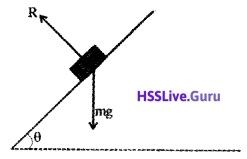1. Which component of weight brings the body towards the bottom along the plane.
2. Find how much force is required to pull the body along the plane.1. mg Sinθ brings the body downwards
2. When the body moves upwards the frictional force (Fs) acts downwards
Total pulling Force = mg Sinθ + Frictional force (Fs) (u, mgCosθ).

Question 5.
Four person sitting in the back seat of a car at rest, is pushing on the front seat.

1. Does the car move. Why?
3. Long jumpers take a long run before the jump. Why?

1. No. Action and reaction cancel each other.
2. Newtons third law of motion.
3. To get large inertia of motion.

Question 6.
A Cricket player lowers his hands while catching a Cricket ball to avoid injury.

1. What do you mean by impulsive force?
2. Prove impulse – momentum theorem.

1. The forces which acton bodies for short time are called impulsive forces.
Example:

• In hitting a ball with a bat
• In firing a gun

2. F = $$\frac{d p}{d t}$$
F∆t = dp
impulse = change in momentum.

### Plus One Physics Law of Motion Four Mark Questions and Answers

Question 1.
A bead sliding on a wire A moves to C through B as shown in the figure. The bead at A has a speed of200cms1. what is speed at B?
2. To what height will it rise before it returns?
3. Why the ball moves up even after reaching the bottom most point B?

1. mgh = 1/2 mv2
m × 10 × 0.8 = 1/2 mv2
V2 = 2 × 10 × 0.8
V = $$\sqrt{2 \times 10 \times 0.8}$$
V = 4 m/s.

2. 80 cm (if friction is neglected).

3. when the ball reaches at B, the potential energy is converted into kinetic energy. Due to this kinetic energy the ball raises to the point c.

Question 2.
Figure shows a block (mass m1) on a smooth horizontal surface, connected by a thin cord that passes over a pulley to a second block (m2), which hangs vertically.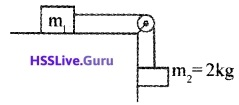1. Obtain formula for the acceleration of the system and tension in the cord.
2. If m1 and m2 interchanges its position, will it affect the tension of the string?
3. What is the acceleration of the system if m1 = 5 kg and m2 = 2kg?

1. When the body m2 moves in down ward direction.
m2g – T = m1 a
T = m2g – m1a.

2. New tension can be found from the relation
m1g – T = m2a
T = m1g – m2 a.

3. Acceleration of system, aQuestion 3.
The collision of two ice hockey players are shown in figure.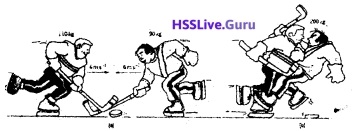Analyse the data given in the figure and answer the following questions.

1. Which conservation law is applicable in this case.
2. In which direction and at what speed do they travel after they stick together.
[Hint – towards right can be taken us +ve direc¬tion and vice versa]
3. If we assume the friction of playing ground is zero, predict the nature of motion and the point at which they come to rest.

1. Conservation of linear momentum.

2. Total momentum before collision = Total momentum after collision.
110 × 4 + 90 × -6 = (110 + 90)v
v = 0.5 m/s
-ve direction, (in the direction of man mass 90 kg).

3. Uniform motion They will not stop.

Question 4.
A circular track of radius 300m is kept with outside of track raised to make 5 degree with the horizontal.

1. Name the process in which outside of the road is raised little above the inner.
2. Obtain an expression for the optimum speed to avoid skidding (considering to friction)

1. Banking of roqd

2.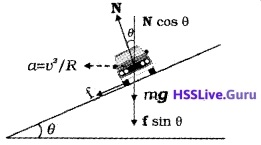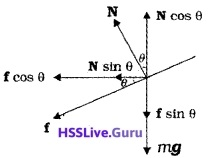Consider a vehicle along a curved road with angle of banking θ. Then the normal reaction on the ground will be inclined at an angle θ with the vertical.
The vertical component can be divided into N Cosθ (vertical component) and N sinθ (horizontal component). The frictional force can be divided into two components. Fcosθ (horizontal component) and F sinθ (vertical component).
From the figure
N cos θ = F sinθ + mg
N cosθ – F sinθ = mg ______(1)
The component Nsin0 and Fsinθ provide centripetal force. Hence
N sinθ + F cos θ = $$\frac{\mathrm{mv}^{2}}{\mathrm{R}}$$ ______(2)
eq (1) by eq (2)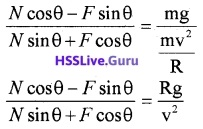Dividing both numerator and denominator of L.H.S by N cosθ. We getThis is the maximum speed at which vehicle can move over a banked curved road.
Optimum speed:
Optimum speed is the speed at which a vehicle can move over a curved banked road without using unnecessary friction. Putting µ = 0 in the above equation we get
v0 = $$\sqrt{\mathrm{Rg} \tan \theta}$$.

Question 5.
A circular track of radius 400m is kept with outer side of track raised to make 5° with the horizontal (coefficient of friction 0.2)
(a) Name such track?
(b) What is optimum speed to avoid wear and tear of type?
(c) What is the maximum permissible speed to avoid skidding?
(a) Banking.

### Plus One Physics Law of Motion Five Mark Questions and Answers

Question 1.
A horse pulls a cart with constant force so that the cart moves with a constant speed.

1. Does it violate Newtons second law of motion?
2. If not, how will you account for the non acceleration of the cart?
3. Will the speed of the cart increase, decrease or remain the same if the horse applied more force?
4. A body of mass 5kg is acted upon by two perpendicular forces 8N and 6N. Give the magnitude and direction of the acceleration of the body.

1. No.

2. The force applied by the car is balanced by the frictional force. Hence the cart moves with constant velocity.

3. If the horse is applied more force, the speed of the cart increases.

4. The resultant force,F = 10N
We know, F = ma
10 = 5 × a
acceleration, a = $$\frac{5}{10}$$ = 2 m / sec2The angle of resultant force,θ = tan-1 6/8
θ = 36°521
The angle of acceleration θ = 36°521.

Question 2.

1. Friction is the force which opposes the relative motion between two surfaces in contact with each other. What is a limiting static friction? State the laws related to this.
2. Show that the coefficient of friction is equal to the tan of the angle between the resultant and normal reactions.
3. For a body of mass 5kg on a plane at a limiting static friction of 30 degrees. What is the force of friction?

1. The maximum value of static friction is called limiting static friction.

• The magnitude of the limiting friction is independent of the area of contact between the surfaces.
• The limiting static friction is directly proportional to the normal reaction R.

ie f α R
fs = µsR.

2. Angle of friction is the angle whose tangent gives the coefficient of friction.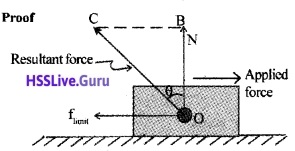Consider a body placed on a surface. Let N be the normal reaction and limit is the limiting friction. Let ‘θ’ be the angle between Resultant vector and normal reaction. From the triangle OBC,∴ tanθ = µ.

3. Tangent of the angle cient of friction.
µs = tanθ
µs = tan 30
µs = $$1 / \sqrt{3}$$
Friction F = µsmg
= $$1 / \sqrt{3}$$ × 5 × 10
F = $$\frac{50}{\sqrt{3}}$$N.

Question 3.
The rate of change of linear momentum of a body is directly proportional to the external force applied on it, and takes place always in the direction of force applied.

1. Name this law.
2. Using this law obtain the expression for force.
3. The motion of a particle of mass m is described by y = ut + $$\frac{1}{2}$$ gt2. Find the force acting on it.

1. Newton’s Second Law.

2. Consider a body of mass ‘m’ moving with a momentum $$\vec{p}$$. Let $$\vec{F}$$ be the force acting on it for time internal ∆t. Due to this force the momentum is changed from $$\vec{p}$$ to p + ∆p. Then according to Newtons second law, we can writeWhere K is a constant pf proportionality. When we take the limit ∆t → 0, we can write3.Hence force F = mg.

Question 4.
Recoil of gun is based on the principle of conservation of momentum.

1. State the principle of conservation of momentum.
2. Explain the reoil velocity of gun.
3. A bullet of mas 100g is fired from a rife of mass 200 kg with a spped of 50 m/s. Calculate the recoil velocity of the rife.

1. According to law of conservation of linear momentum, if the external force acting on a body is zero, total linear momentum remains constant. According to Newton’s second law.
F = $$\frac{d p}{d t}$$
If F = 0, $$\frac{d p}{d t}$$ = 0 i.e; P is constant.

2. Let M, m be the mass of gun and bullet respectively. Let V and ν be the velocities of gun and bullet after firing.
According to consevation of momentum
Total momentum before firing = Total momentum after firing
∴ O = MV + m ν
-MV = mν
The above equation shows that when bullet moves in forward direction, the gun moves in back direction. This motion of gun is called recoil of gun.

3. M = 200kg, m = 100g = 0.1kg
ν = 50 m/s, V = ?
MV = mν
200 × V = 0.1 × 50
V = $$\frac{0.1 \times 50}{200}$$m/s.

Question 5.
While firing a bullet, the gun must be held tight to the shoulder.

1. Which conservation law helps you to explain this
2. “In the firing process, the speed of the gun is very low compared to the speed of the bullet.” Substantiate the above statement using mathematical expressions.
3. A shell of 20kg moving at 50m/s bursts in to two parts of masses 15kg and 5kg. If the larger part continues to move in the same direction at 70 m/s. What is the velocity and direction of motion of the other piece.

1. Conservation of momentum.

2. Total momentum is conserved
∴ mu + MV = 0
V = $$\frac{-m u}{M}$$ M is very large. Hence v is small

3. MV = m1 u1 + m2 u2
20 × 50 = 5u1 + 15 × 70
5u1 = 50
u1 = 10m/s.

Question 6.
While firing a bullet, the gun must be held tight to the shoulder.

1. This is a consequence of______
2. Show that recoil velocity is opposite to the muzzle velocity of the bullet.
3. A gun of mass 5 kg fire a bullet of mass 5g, vertically upwards to a height of 100m. Calculate the recoil velocity of gun.

1. Conservation of linear momentum.

2. Let M be the mass of gun and m be the mass of bullet. When gun fires, the gun and bullet acquire velocities V and v respectively.
According to conservation of momentum.
Total momentum before firing = Total momentum afterfiring
m × o + M × o = mu + MV
O = mv + MV
ie. – MV = mv
V = $$\frac{-m v}{M}$$

3. M = 5kg, m = 5 × 10-3 kg, h = 100m
v2 = u2 + 2as
0 = u2 + 2 × 10 × 100
Velocity of bullet,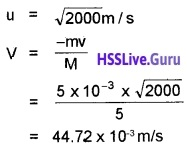Question 7.
A standing passenger falls backwards when the bus starts suddenly.

1. Explain why this happens?
2. Which Newtons law gives the above concept. State the law.
3. Obtain an expression for force using Newtons law.

1. Due to inertia of rest, the body continues in the state of rest.

2. Newtons first law:
Everybody continues in its state of rest or of uniform motion along a straight line unless it is compelled by an external unbalanced force to change that state:

3. Consider a body of mass ‘m’ moving with a momentum $$\vec{p}$$. Let $$\vec{F}$$ be the force acting on it for time internal ∆t. Due to this force the momentum is changed from $$\vec{p}$$ to p + ∆p. Then according to Newtons second law, we can writeWhere K is a constant pf proportionality. When we take the limit ∆t → 0, we can writeQuestion 8.
According to Newton’s law of motion rate of change of momentum is directly proportional to applied force.
a. Impulse has the unit similarto that of

1. Momentum
2. force
3. time
4. Energy

b. A man falling from certain height receives more injuries when he falls on a marble floor than when he falls on a heap of sand. Explain. Why?
c.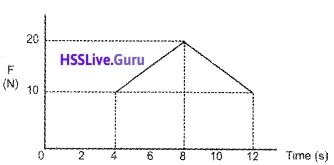Force – time graph for a body starting from rest is shown in the figure. What is the velocity of the body at the end of 12 second? (Mass of the body is 5 kg)
a. 1. Momentum.

b. When a man falls on a marble floor, the momentum is reduced to zero in lesser time. Due to this, the rate of change of momentum is large. So greater force acts on a man falls on marble floor.

c. The area of force – time graph gives change in momentum.
ie. change in momentum,
mv = 1/2 × (12 – 4) × (20 -10)
mv = 40

### Plus One Physics Law of Motion NCERT Questions and Answers

Question 1.
Give the magnitude and direction of the net force acting on
(a) a drop of rain falling down with a constant speed.
(b) a cork of mass 10g floating on water
(c) a kite skillfully held stationary in the sky
(d) a car moving with a constant velocity of 30km h-1 on a rough road
(e) a high – speed electron in space far from all material objects, and free of electric and magnetic fields.
Applying Newton’s first law of motion, we find that no net force acts in any of the situations, (a) to (d). Again, no force in situation (e). This is because electron is far away from all material agencies producing electromagnetic and gravitational forces.

Question 2.
A constant retarding force of 50 N is applied to a body of mass 20kg moving initially with a speed of 15ms-1. How long does the body take to stop?
Acceleration, a = –$$\frac{50 \mathrm{N}}{20 \mathrm{kg}}$$ = -2.5ms-2
u = 15ms-1, v = 0, t = ?
v = u + at
0 = 15 – 2.5t or 2.5t = 15 or
t = $$\frac{15}{2.5}$$s = 6.0s.

Question 3.
A constant force acting on a body of mass 3.0kg changes its speed from 2.0ms-1 to 3.5 ms-1 in 25s. The direction of motion of the body remains unchanged. What is the magnitude and direction of the force?
m = 3kg; u = 2ms-1; v = 3.5 ms-1;
t = 25s ; F = ?
v = u + at
3.5 = 2 + 25a or a = 0.06 ms-2
F = ma = 3kg × 0.06 ms-2 = 0.18N.
The direction of force is along the direction of motion.

Question 4.
A bob of mass 0.1 kg hung from the ceiling of a room by a string 2 m long is set into oscillation. The speed of the bob at its mean position is 1 ms-1. What is the trajectory of the bob if the string is cut when the bob is

1. at one of its extreme positions.
2. at its mean position.

1. At the extreme position, the speed of the bob is zero. If the string is cut, it will fall vertically down wards.
2. At the mean position, the bob has a horizontal velocity. If the string is cut, it will fall along a parabolic path.

Question 5.
A man of mass 70kg stands on a weighing scale in a lift which is moving

1. upwards with a uniform speed of 10ms-1
2. downwards with a uniform acceleration of 5ms-2
3. upwards with a uniform acceleration of 5ms-2 What would be the readings on the scale in each case?
4. What would be the reading if the lift mechanism failed and it hurtled down freely under gravity?

1. a = 0, R = mg = 70 × 10 N = 700N
2. mg – R = , ma ; R – mg – ma = (g – a)
= 70(10 – 5) N = 350N
3. R – mg = ma or R = m(g + a)
= 70(10 + 5)N = 1056 N
4. In the event of free fall, it is a condition of weight lessness.

Question 6.
A nucleus is at rest in the laboratory frame of reference. Show that if it dist integrates into two smaller nuclei, the products must move in opposite directions.